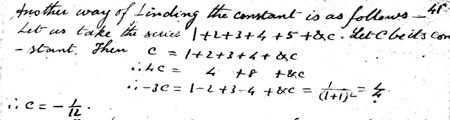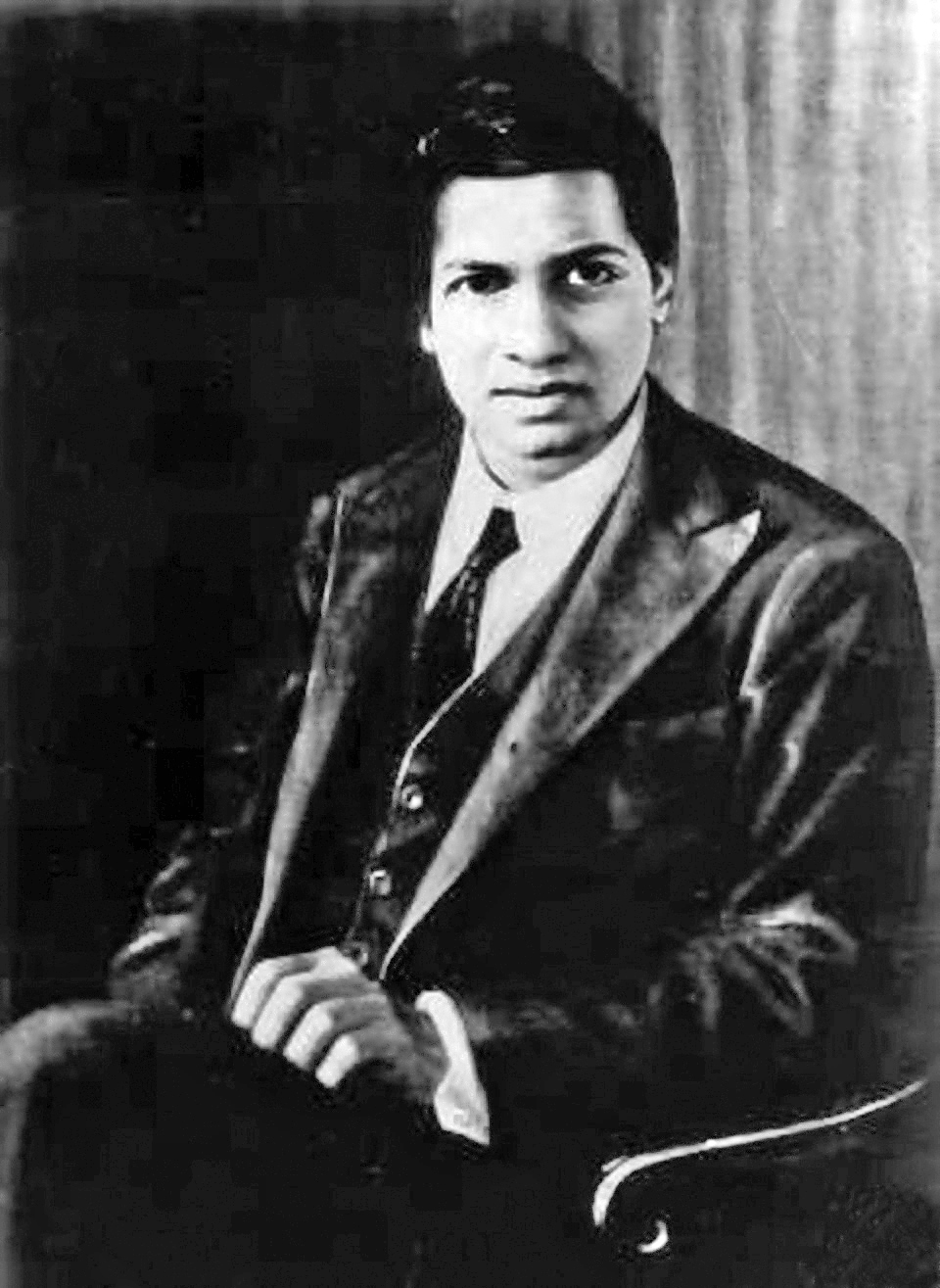# Convergence of a Divergent Series and other Tests

In class we have been toying with the idea of classifying diverging infinite series, such as the sum: Σ k (k = 1,) = 1 + 2 + 3 + … which, as we add it up, continues on to infinity. We also messed around with some series notations and came to a conclusion that the sum adds up to -1/12. Now, I have no intention to claim, nor prove, that it equals -1/12. In fact, I would like to do the opposite; I would like to show that it in no way converges. If an infinite series converges, that means it sums up to a real number s: Σ ak = s. Likewise, an infinite series diverges if the sum of the series equals ±∞ or it does not add to any value. There are a few methods I will use to prove that this series diverges, and these methods can also be used to determine whether any infinite series converges or diverges.

The first test I want to look at is the Root Test. In this test, we take the sequence ak raise it to the power 1/k, and take the limit of that as k→∞. If this limit is greater than 1, the series diverges; if the limit is less than 1, it converges. For the sum of all integers, that gives us limit as k→∞ of k(1/k) . As k→∞, limit of k(1/k) → 1. Unfortunately the limit equals 1, which means it is inconclusive; there is a chance that this does indeed sum up to -1/12. Let’s leave the score at 0-0.

I will now put the series through the Ratio Test. It is called the Ratio Test because we take a ratio of the k+1 term divided by the k-th term and take the limit of that as k→∞: the limit of ak+1/ak. Similar to the Root Test, if the limit is greater than one it diverges, and if the limit is less than 1 it converges. For the dubious series which is under examination, that limit (k+1)/k, and as k→∞ the limit equals 1. Once again, this test is inconclusive. The score is still 0-0.

Now it’s time to get serious; so far every test I’ve done has been disappointingly empty of an answer. In this test if a series bk > ak and bk converges, then ak must also converge and if bk < ak and bk diverges, then ak diverges as well. My claim is that Σ k (k = 1,∞) diverges and now I will compare it with a series Σ 1 (k = 1,n) = n. We can see that 1+1+1+… grows much more slowly than 1+2+3+… thus we can use it as a comparison. By another test, the Term Test, if the series converges then lim an = 0. The series must diverge then if the limit doesn’t equal 0. The limit of 1 as n→∞ = 1 ≠ 0, thus the sum of 1 on (k=1,∞) diverges. Since Σ 1 (k = 1,∞) < Σ k (k = 1,∞), the latter series must also diverge. Now the score is 1 for divergence, and 0 for -1/12.

While it can now be seen that Σ k (k = 1,∞) does indeed diverge, the comparison test relies on having knowledge that a similar infinite series will either converge or diverge. In this case, I have to know that Σ 1 (k = 1,∞) diverges before I can compare it to the original series. I used the Term Test to show that Σ 1 (k = 1,∞) diverged, but I can also use it to show that Σ k (k = 1,∞) diverges. The limit as n→∞ of k = ∞, and by the Term Test diverges. The problem with the Term Test is that the limit ak can be equal to zero, but the series can still diverge. Therefore, this test is only useful if the limit does not equal 0 or to insure that a converging series does indeed converge.

By both the Term and Comparison tests, I was able to show that Σ k (k = 1,∞) diverges and is not equal to -1/12. In class though, we determined that the infinite series itself doesn’t sum to a negative number, there is no possible way that adding large positive whole numbers together would result in a negative rational number, but rather -1/12 represented a value or categorization of the infinite sum Σ k (k = 1,∞). How did this number come about? The idea is definitely not of “fringe mathematics” and has some excellent arguments. The idea stems from Σ (-1)k (k = 0,∞) = 1 – 1 + 1 – 1 + … The mathematician Srinivasa Ramanujan gave this sum a “value” of ½, since it seems to jump between one and zero equally, with ½ as the average.

The second sum Σ k(-1)k-1 (k = 1,∞) = ¼ as this sum added to itself equals Σ (-1)k (k = 0,∞). That is 1 – (2 – 1) + (3 – 2) + … = 1 – 1 + 1 – 1 + … Finally, Σ k (k = 1,∞) – 4(Σ k (k = 1,∞)) = 1 + (2 – 4) + 3 – (4 – 8) + … equals 1 – 2 + 3 – 4 + … = Σ k(-1)k-1 (k = 1,∞), or rather -3(Σ k (k = 1,∞)) = ¼ Σ k (k = 1,∞) = -1/12.Ramanujan discovering the value -1/12 for the the infinite series ak = k. Image: Srinivasa Ramanujan via Wikimedia Commons.

The specific stipulation given is that the series must go to its limit. The partial summations of any of these series will produce a number unlike the total summation. For the majority of mathematics, to say Σ k (k = 1,∞) = ∞ makes more sense and requires significantly less head-scratching than -1/12.

There is one more test for convergence that I did not talk about, as the infinite series I was examining did not apply, and that is the Integral Test. For Σ ak, the function f(k) = ak. The reason why I could not apply it is because it only works with positive, non-increasing functions bounded on [1,∞). If the integral of f(x) is a real number, then the series converges, whereas if that same integral diverges, then the series diverges. The first case I will look at is Σ 1/k (k = 1,∞), where f(x) = 1/x and is a positive, non-increasing function. The integral of 1/x = log(x) evaluated from 1 to ∞. This integral turns out to diverge, and therefore the series also diverges. The second case is similar: Σ 1/k2 (k = 1,∞), where f(x) = x-2. The integral of 1/x2 = -(1/x) evaluated over the same interval. The sum of these two bounds is 0 – (-1) = 0 + 1 = 1. Since the indefinite integral converges, the series also converges.

These tests help determine whether a series converges or not. I used them to prove with basic mathematics that Σ k (k = 1,) diverges, rather than converging on a negative rational number. While the values given to divergent indefinite series can provide an idea of how they relate to each other, they require a fair amount of assumption and a lot of counterintuitive work to calculate. It is far easier and more practical to state that Σ k (k = 1,) diverges.

Sources:

http://www.lemiller.net/media/classfiles/notes.pdf (Foundations of Analysis by Joseph L. Taylor)

https://www.math.hmc.edu/calculus/tutorials/convergence/

http://mathworld.wolfram.com/RatioTest.html

http://en.wikipedia.org/wiki/Series_%28mathematics%29

http://en.wikipedia.org/wiki/1_%2B_2_%2B_3_%2B_4_%2B_%E2%8B%AF

http://en.wikipedia.org/wiki/Srinivasa_Ramanujan

# Marveling at the Indian Genius: Ramanujan

Hardy left the cab. He was visiting an ill Ramanujan at Putney. Ramanujan asked about his trip. Hardy remarked the ride was dull; even the taxi number, 1729, was dull to the number theorist. He hoped the number’s dullness wasn’t an omen predicting Ramanujan’s declining health. “No Hardy,” Ramanujan replied, “it is a very interesting number.” The integer 1729 is actually the smallest number expressible as the sum of two positive cubes in two different ways. Indeed 1729 = 13 + 123 = 93 + 103.Ramanujan. Picture courtesy of Konrad Jacobs. From Wikimedia Commons

The mathematicians Srinivasa Iyengar Ramanujan and G.H. Hardy comprise the characters of this story. Ramanujan’s casual discovery of the smallest so-called taxicab number was no fluke. Ramanujan and Hardy’s most famous result was an asymptotic formula for the number of partitions of a positive integer. A partition of a number n is a way of writing n as the sum of positive integers. Reordering terms doesn’t change a partition. Thus the partitions of 5 are 5, 4 + 1, 3 + 2, 3 + 1 + 1, 2 + 1 + 1 + 1, and 1 + 1 + 1 + 1 + 1; hence 5 has 7 seven partitions. Counting the partitions becomes difficult as n grows. Ramanujan had made several conjectures based on numerical evidence, and Hardy credits many of the needed insights to Ramanujan. The formula is complicated and counter-intuitive. It involves values of √3, π, and e, all very strange numbers for a counting formula. Near his death, Ramanujan discovered mock theta functions, which mathematicians are still “rediscovering” today.

These are only the achievements of later Ramanujan. The early life of Ramanujan is even more surprising. He spent his childhood in the poor south Indian town, Kumbakonam. In school, he scored top marks. He probed college students for mathematical knowledge by age 11. When he was 13, he comprehended S.L. Loney’s advanced trigonometry book. He could solve cubic and quartic equations—the latter method he found himself—and finished math tests in half the allotted time. If asked, he could recite the digits of π and e to any number of digits. He borrowed G.S. Carr’s A Synopsis of Elementary Results in Pure and Applied Mathematics by age 16 and worked through its many theorems. By 17, he independently investigated the Bernoulli numbers, a set of numbers intimately connected to number theory.

So How did Ramanujan do it? No brilliant teacher—excluding Hardy and J.E. Littlewood when Ramanujan was already an adult—taught Ramanujan. The odds were against Ramanujan from the start. Ramanujan often used slate instead of paper because paper was expensive; he even erased slate with his elbows, since finding a rag would take too long. He lost his first college scholarship by neglecting his every subject that wasn’t math. The first two English mathematicians he sent letters to request publication did not respond; was it luck that Hardy did?

Ramanujan credits Namagiri, a family deity, for his mathematics. He claimed the goddess would write mathematics on his tongue, that dreams and visions would reveal the secrets of math to him.

Ramanujan was undoubtedly religious. Before leaving India, he respected all the holy customs of his caste: he shaved his forehead, tied his hair into a knot, wore a red U with a white slash on his forehead, and refused to eat any meat. In the Sarangapani temple, Ramanujan would work on his math in his tattered notebook.

Hardy, the ardent atheist, didn’t believe that gods communicated with Ramanujan. He thought flashes of insight were just more common in Ramanujan than in most other mathematicians. Sure, Ramanujan had his religious quirks, but they were just quirks.

Looking at Ramanujan’s work, I find it hard to deny that some god aided the man. I cannot even imagine Ramanujan’s impact had he lived longer or had better teachers.

Sources and cool stuff:

biographical stuff: http://www-history.mcs.st-andrews.ac.uk/Biographies/Ramanujan.html, Christopher Syke’s documentary Letter’s from and Indian Clerk, Robert Kanigel’s The Man Who Knew Infinity

Ramanujan and Hardy’s original paper on partitions: http://plms.oxfordjournals.org/content/s2-17/1/75.full.pdf

Wolfram on taxicab numbers: http://mathworld.wolfram.com/TaxicabNumber.html

Wolfram on Hardy-Ramanujan number: http://mathworld.wolfram.com/Hardy-RamanujanNumber.html

Wolfram on Bernoulli numbers: http://mathworld.wolfram.com/BernoulliNumber.html

Ramanujan’s papers and notebooks: http://www.imsc.res.in/~rao/ramanujan/contentindex.html

mock theta functions: http://www.ams.org/notices/201011/rtx101101410p.pdf